# 24. Moving Watermarks Video with Python

The idea behind this chapter of our Python course is to create a film from one or more pictures where one or more object in form of watermarks are moved around.

We will move around solid objects or shining-through watermark objects. We created an image with a watermark structure for our chapter on decorators and decoration. You can see it close to the top of the page: An "ampersand" (better known in the Python community as the decortor sign. You can also find a course on how to create watermarked images like this with Python, Numpy, Scipy and Matplotlib in the chapter on Image Processing Techniques. It explains the whole process of the making-of of our decorator and at sign picture, which means watermarked images. So consulting these chapters before going on may be a good idea. Technical note: opencv

## Creating Interesting Objects with Matplotlib

We start by creating some nice objects which we can use in the film we want to create as moving objects. We start by creating a directory to hold the objects:

import os
import shutil
target_dir = 'images4video'
watermarks_dir = f"{target_dir}/watermarks_dir"
if os.path.exists(watermarks_dir):
# delete the existing directory
shutil.rmtree(watermarks_dir)
# Create target_dir, because it doesn't exist so far
os.makedirs(watermarks_dir)


We use in the following code the parameter bbox_inches="tight" in savefig. When this parameter is set to "tight", matplotlib will try to remove any extra whitespace or padding around the edges of the figure, so that only the actual content of the figure is saved. This can be useful when you want to save a figure with minimal margins or padding.

import numpy as np
import matplotlib.pyplot as plt

x = np.linspace(-2, 2, 1000)
y1 = np.sqrt(1-(abs(x)-1)**2)
y2 = -3 * np.sqrt(1-(abs(x)/2)**0.5)

fig, ax = plt.subplots()

ax.fill_between(x, y1, color='red')
ax.fill_between(x, y2, color='red')
ax.axis(xmin=-2.3, xmax=2.3)
ax.axis('off')
#ax.xlim([-2.5, 2.5])
txt2include = "python-course.eu"
ax.text(0, -0.4,
txt2include,
fontsize=24,
fontweight='bold',
color='orange',
horizontalalignment='center')

plt.savefig(f"{watermarks_dir}/heart.png", bbox_inches="tight")We create now a an image with a black and white spiral:

import numpy as np
import matplotlib.pyplot as plt

r = theta**2
x_2 = r*np.cos(theta)
y_2 = r*np.sin(theta)
plt.figure(figsize=[5, 5])
plt.gcf().set_size_inches(10, 10)
plt.plot(x_2, y_2, linewidth=50.5, color='black')
plt.axis('off')
plt.savefig(f"{watermarks_dir}/spiral.png", bbox_inches="tight")
plt.show()What about colours? A different spiral but now in colours:

import matplotlib.pyplot as plt
import numpy as np

theta = np.arange(0, 8*np.pi, 0.1)
a, b = 1, 0.5

for dt in np.arange(0, 2*np.pi, np.pi/2.0):
x = a*np.cos(theta + dt)*np.exp(b*theta)
y = a*np.sin(theta + dt)*np.exp(b*theta)
dt = dt + np.pi/4.0
x2 = a*np.cos(theta + dt)*np.exp(b*theta)
y2 = a*np.sin(theta + dt)*np.exp(b*theta)
xf = np.concatenate((x, x2[::-1]))
yf = np.concatenate((y, y2[::-1]))
p1 = plt.fill(xf, yf)

plt.axis('equal')
plt.axis('off')
plt.tight_layout()
plt.savefig(f'{watermarks_dir}/coloured_spiral.png', bbox_inches="tight")Live Python training

Enjoying this page? We offer live Python training courses covering the content of this site.

Enrol here

## Size of an Image

The following function returns the size of an image as a tuple (width, height). opencv has to be installed so that you can use the cv2 module

import cv2

def get_frame_size(image_path):
""" Reads an image and calculates
the width and length of the images,
which will be returned """
height, width, layers = frame.shape
frame_size = (width, height)
return frame_size

get_frame_size(f"{watermarks_dir}/spiral.png")


### OUTPUT:

(794, 790)


## Random Tile in Image

Now, we define a function random_tile which returns the coordinates (top left corner and bottom right corner) of a tile in the image img. The size of this tile os equal to the size of the watermark image which is passed to the function:

import random

def random_tile(img, watermark_image):
""" returns the top left and bottom right corner
of a random tile in an image. The size correspondents to
the size of the watermake image """
height, width, colours = img.shape
w_height, w_width, colours = watermark_image.shape
tile_upper_left_row = int(round(random.randint(0, height - w_height), 0))
tile_upper_left_column = int(round(random.randint(0, width - w_width), 0))
top_left = tile_upper_left_row, tile_upper_left_column
bottom_right_row = int(round(tile_upper_left_row + w_height, 0))
bottom_right_column = int(round(tile_upper_left_column + w_width, 0))
bottom_right = bottom_right_row, bottom_right_column


We will use cv2.imread to read in an image, which we want to use in our examples. We could also use cv2.imshow, but this opens an external window in the Jupyter-Notebook, which we use to create this website. This is the reason, why we use plt.imshow to view the image. We have to mirror the colours before we can use plt.imshow, because by using cv2.imread, we got a BGR (blue, green, red) image, whereas plt.imread returns RGB-Images.

import matplotlib.pyplot as plt
import random
import numpy as np
import cv2

rgb_img = image[:, :, ::-1]  # BGR ---> RGB
#plt.axis('off')
plt.imshow(rgb_img)


### OUTPUT:

<matplotlib.image.AxesImage at 0x7f12bfe432d0>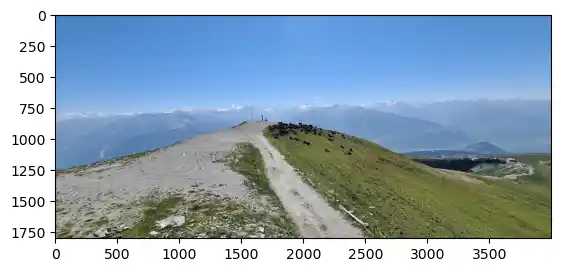spiral = cv2.imread(f"{watermarks_dir}/spiral.png")
rgb_spiral = spiral[:, :, ::-1]  # BGR ---> RGB
plt.imshow(rgb_spiral)


### OUTPUT:

<matplotlib.image.AxesImage at 0x7f12aed82150>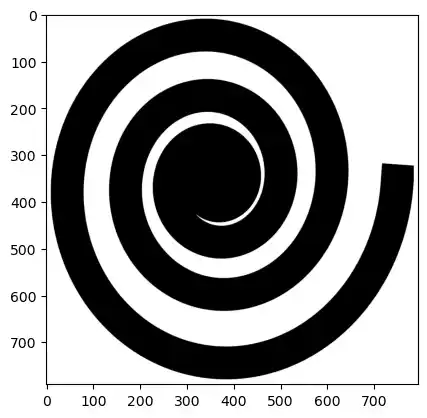We test our function random_tile with these two images:

top_left, bottom_right = random_tile(image, spiral)
print(f"{top_left=}, {bottom_right=}")
print(f"{spiral.shape=}, {image.shape=}")


### OUTPUT:

top_left=(501, 2217), bottom_right=(1291, 3011)
spiral.shape=(790, 794, 3), image.shape=(1800, 4000, 3)


We can see that the dimensions of the random tile correspond to the watermark image:

height_of_tile = bottom_right - top_left
width_of_tile = bottom_right - top_left
print( (height_of_tile, width_of_tile) == spiral.shape[:2])


### OUTPUT:

True


import matplotlib.pyplot as plt
import random
import numpy as np
import cv2

rgb_img2 = image2[:, :, ::-1]  # BGR ---> RGB
plt.imshow(rgb_img2)


### OUTPUT:

<matplotlib.image.AxesImage at 0x7f12aec232d0>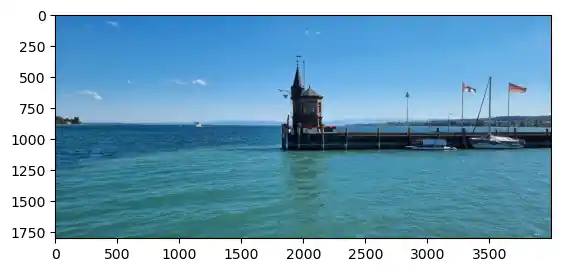Live Python training

Enjoying this page? We offer live Python training courses covering the content of this site.

Upcoming online Courses

Enrol here

With the aid of the function watermark_tile, we can put a watermark on the area of the tile defined by the parameters top_left, bottom_right. The watermark is supposed to be black and white. Where the watermark is black, the corresponding pixels from imag2 are taken and were they are white the pixels from imag1 are taken.

def watermark_tile(imag1, imag2, watermark_imag, top_left, bottom_right):
""" The pixels of imag2 are used when the watermark_imag is black """
r1, r2 = top_left, bottom_right
c1, c2 = top_left, bottom_right
tile = imag1[r1:r2, c1:c2]
tile[:] = np.where(watermark_imag>(1, 1, 1), imag1[r1:r2, c1:c2], imag2[r1:r2, c1:c2])


We test this function now with the images 'image', 'image2' and 'spiral' as the watermark image:

watermark_tile(image, image2, spiral,  top_left, bottom_right)

rgb_img = image[:, :, ::-1]  # BGR ---> RGB
plt.imshow(rgb_img)


### OUTPUT:

<matplotlib.image.AxesImage at 0x7f12aec432d0>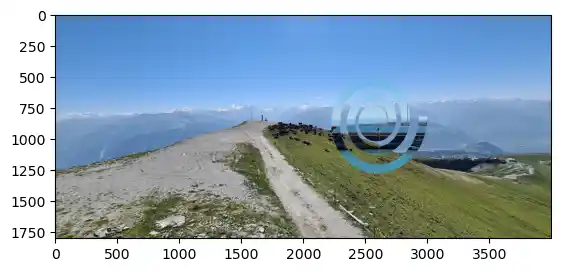## Watermark on tile

Now we want to put a watermark image directly on an image 'imag1'. We use a coloured image as a watermark.

def object_on_tile(imag1, object_image, top_left, bottom_right):
""" The object is put over the image """
r1, r2 = top_left, bottom_right
c1, c2 = top_left, bottom_right
#print(f'{object_image=}')
#tile = imag1[r1:r2, c1:c2]
imag1[r1:r2, c1:c2] = np.where(object_image>(250, 250, 250),
imag1[r1:r2, c1:c2],
object_image)

watermark_image = cv2.imread(f"{watermarks_dir}/coloured_spiral.png")
rgb_watermark_image = watermark_image[:, :, ::-1]  # BGR ---> RGB
plt.imshow(rgb_watermark_image)


### OUTPUT:

<matplotlib.image.AxesImage at 0x7f12aeb0b410>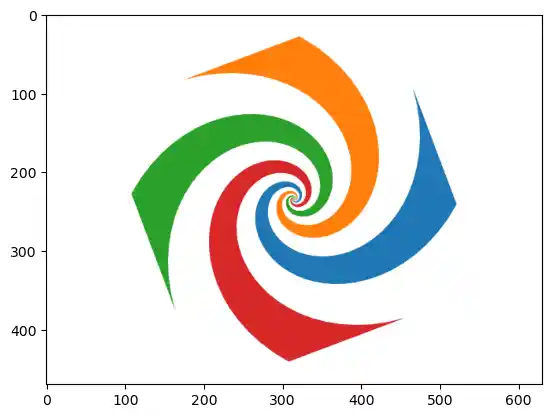watermark_image.shape


### OUTPUT:

(470, 630, 3)

image = cv2.imread(f"{target_dir}/bodensee_area/boat.jpg")
plt.imshow(image[:, :, ::-1])


### OUTPUT:

<matplotlib.image.AxesImage at 0x7f12aebb0390>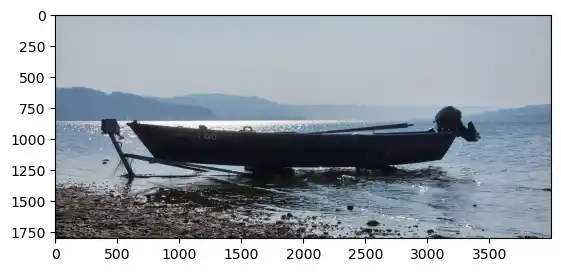top_left, bottom_right = random_tile(image, watermark_image)

object_on_tile(image, watermark_image,  top_left, bottom_right)

rgb_img = image[:, :, ::-1]  # BGR ---> RGB
plt.imshow(rgb_img)


### OUTPUT:

<matplotlib.image.AxesImage at 0x7f12aea24d50>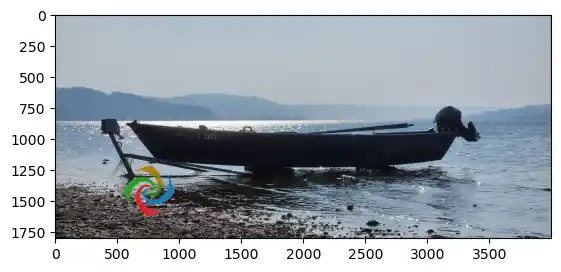image.shape


### OUTPUT:

(1800, 4000, 3)

watermark_image = plt.imread(f"{watermarks_dir}/spiral.png")
watermark_image = watermark_image[:, :, :3]
plt.imshow(watermark_image)
#cv2.imshow(image)


### OUTPUT:

<matplotlib.image.AxesImage at 0x7f12aeaaebd0>Live Python training

Enjoying this page? We offer live Python training courses covering the content of this site.

Enrol here

## Tile Close to Another

We write now a function new_close_tile, which randomly finds a new tile close to an existing tile in the vicinity random.

import random

def new_close_tile(top_left, bottom_right,
img_width, img_height,
""" finds randomly a new tile close to the given tile
row1, row2 = top_left, bottom_right
col1, col2 = top_left, bottom_right
tile_height = row2 - row1
tile_width = col2 - col1
row1_new, row2_new = row1 + y, row2 + y
col1_new, col2_new = col1 + x, col2 + x
while (row2_new > img_height) or row1_new < 0 or col1_new < 0 or col2_new > img_width:
row1_new, row2_new = row1 + y, row2 + y
col1_new, col2_new = col1 + x, col2 + x
return ((row1_new, col1_new), (row2_new, col2_new))


We will test now the function new_close_tile.

# find a tile:
top_left, bottom_right = random_tile(image, watermark_image)
print(f"{top_left=} {bottom_right=}")
img_height, img_width, colours = image.shape
for i in range(5):
top_left, bottom_right = new_close_tile(top_left, bottom_right,
img_width, img_height,
print(f"New tile positions: {top_left=} {bottom_right=}")


### OUTPUT:

top_left=(222, 1780) bottom_right=(1012, 2574)
New tile positions: top_left=(226, 1779) bottom_right=(1016, 2573)
New tile positions: top_left=(226, 1783) bottom_right=(1016, 2577)
New tile positions: top_left=(226, 1783) bottom_right=(1016, 2577)
New tile positions: top_left=(223, 1787) bottom_right=(1013, 2581)
New tile positions: top_left=(218, 1787) bottom_right=(1008, 2581)


## Images with Moving Watermarks

n = 120
watermark_pics = f'{target_dir}/watermark_pics'
if os.path.exists(watermark_pics):
shutil.rmtree(watermark_pics)
os.makedirs(watermark_pics)
watermark_image = watermark_image[:, :, :3]
height, width, colours = image_orig.shape
top_left, bottom_right = random_tile(image, watermark_image)
print(top_left, bottom_right)
for i in range(n, 0, -1):
image = image_orig.copy()
top_left, bottom_right = new_close_tile(top_left, bottom_right, width, height, 20)
object_on_tile(image, watermark_image, top_left, bottom_right)
cv2.imwrite(f"{watermark_pics}/pic_{i:03d}.png", image)


### OUTPUT:

(1052, 3352) (1522, 3982)

def video_from_images(folder='.',
video_name = 'video.avi',
suffix='png',
prefix='pic_',
reverse=False,
length_of_video_in_seconds=None,
codec = cv2.VideoWriter_fourcc(*'DIVX')):
""" The function creates a video from all the images with
the suffix (default is 'png' and the prefix (default is 'pic_'
in the folder 'folder'. If 'length_of_video_in_seconds' is set
to None, it will be the number of images in seconds. If a positive
value is given this will be the length of the video in seconds.
The function assumes that the the shape of the first image is
the one for all the images. If not a warning will be printed
and the size will be adapted accordingly! """

images = []
for fname in glob.glob(f'{folder}/{prefix}*{suffix}'):
images.append(fname)
images.sort(reverse=reverse)
if length_of_video_in_seconds is None:
# each image will be shown for one second
length_of_video_in_seconds = len(images)

# calculate number of frames per seconds:
frames_per_second = len(images) / length_of_video_in_seconds
frame_size = get_frame_size(images)

video = cv2.VideoWriter(video_name,
codec,
frames_per_second,
frame_size)

for image in images:
height, width, layers = im.shape
if (width, height) != frame_size:
print(f'Warning: {image} resized from {(width, height)} to {frame_size}')
im = cv2.resize(im, frame_size)
video.write(im)
cv2.destroyAllWindows()
video.release()


We have 120 pictures created and we create now a video with a length of 10 secondes which makes 12 pictures per second:

import glob
video_from_images(watermark_pics,
f'{target_dir}/colour_spiral.avi',
'png',
length_of_video_in_seconds = 10)


For the next video, we will use our black and white spiral. So we will load it first:

watermark_image = cv2.imread(f"{watermarks_dir}/spiral.png")
plt.imshow(watermark_image[:,:,::-1])


### OUTPUT:

<matplotlib.image.AxesImage at 0x7f12ae936bd0>n = 40
watermark_pics = f'{target_dir}/watermark_pics2'
if os.path.exists(watermark_pics):
shutil.rmtree(watermark_pics)
os.makedirs(watermark_pics)

imag_tinted = image_orig // 2
for i in range(n, 0, -1):
image = image_orig.copy()
top_left, bottom_right = random_tile(image, watermark_image)
watermark_tile(image, imag_tinted, watermark_image,  top_left, bottom_right)
cv2.imwrite(f"{watermark_pics}/pic_{i:03d}.png", image)

import glob
video_from_images(watermark_pics,
f'{target_dir}/spiral.avi',
'png',
length_of_video_in_seconds=40)


You can listen to the videos we created in this tutorial:

I used this technique to create a video for a music composition of mine. The video can be found at YouTube:

Music piece for piano, saxophone, oboe and drums I added the sound track with the Linux program kdenlive.

You can find all my videos including a Python video at Bernd Klein's Videos

Live Python training

Enjoying this page? We offer live Python training courses covering the content of this site.

Upcoming online Courses

Enrol here